•Gurobi Staff

Hi Jose,

It seems like you already have the 1st and 2nd degree polynomials for the domains $$y > 120x$$ and $$y \leq 120x$$, which is very good and you only need to focus on modeling the conditional constraint. What you have given is

\begin{align} \min\,\,&obj\\ d_1 &= \text{1st degree polynomial}(x,y)\\ d_2 &= \text{2nd degree polynomial}(x,y) \end{align}
where $$obj$$ is an auxiliary objective variable and $$d_1,d_2$$ are auxiliary optimization variables. They are used to make the modeling of the conditional statement easier.

Now what you are looking for is
$$\texttt{if}$$ $$y > 120x$$ $$\texttt{then}$$ $$obj = d_2$$ $$\texttt{else}$$ $$obj = d_1$$.

A detailed description on modeling of conditional statements, such as the one above, is described in the Knowledge Base article How do I model conditional statements in Gurobi?

Best regards,
Jaromił

•Hi Jaromil,

Thanks so much for your prompt response. :)

I found out that the fit is even better if I create more than 2 piecewise objectives like below.

if (I <= 120*SOC_avg)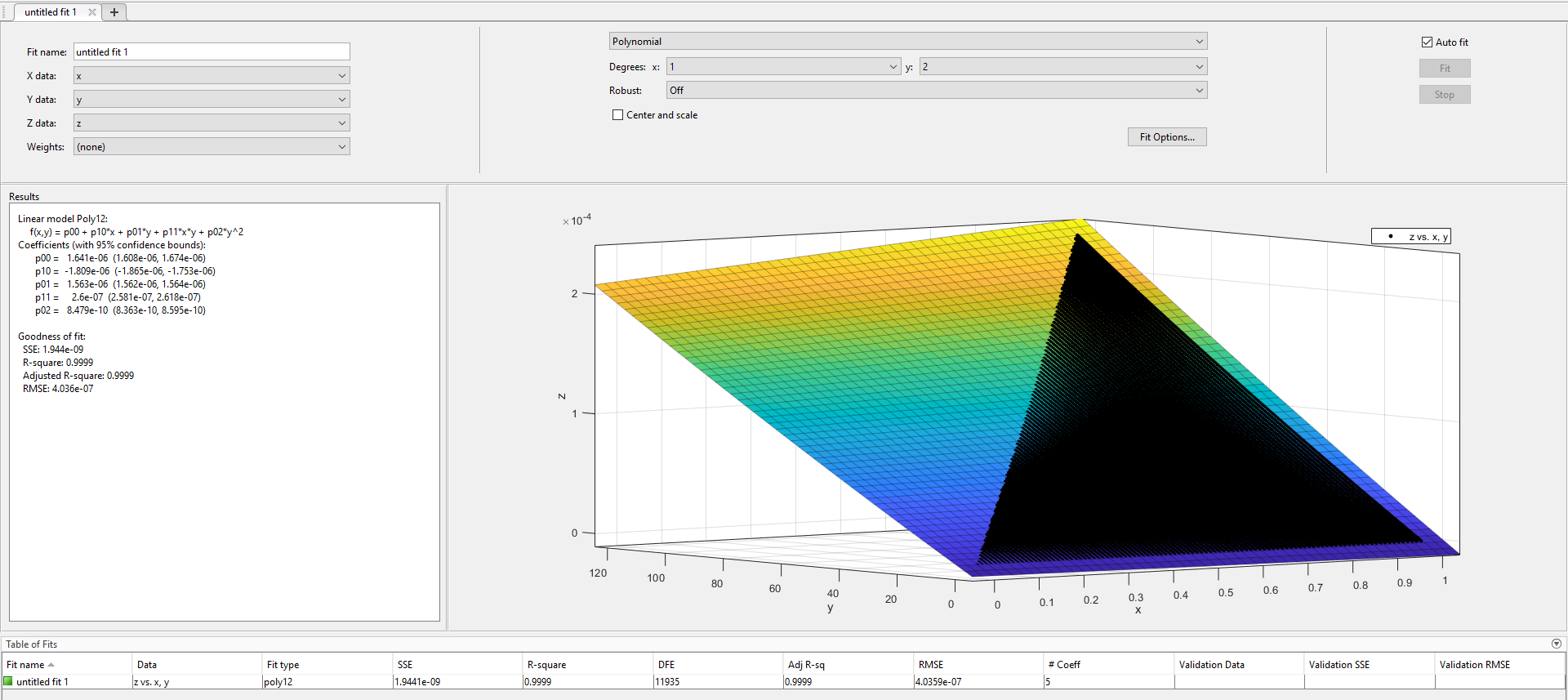if (I > 120*SOC_avg and <= 240*SOC_avg)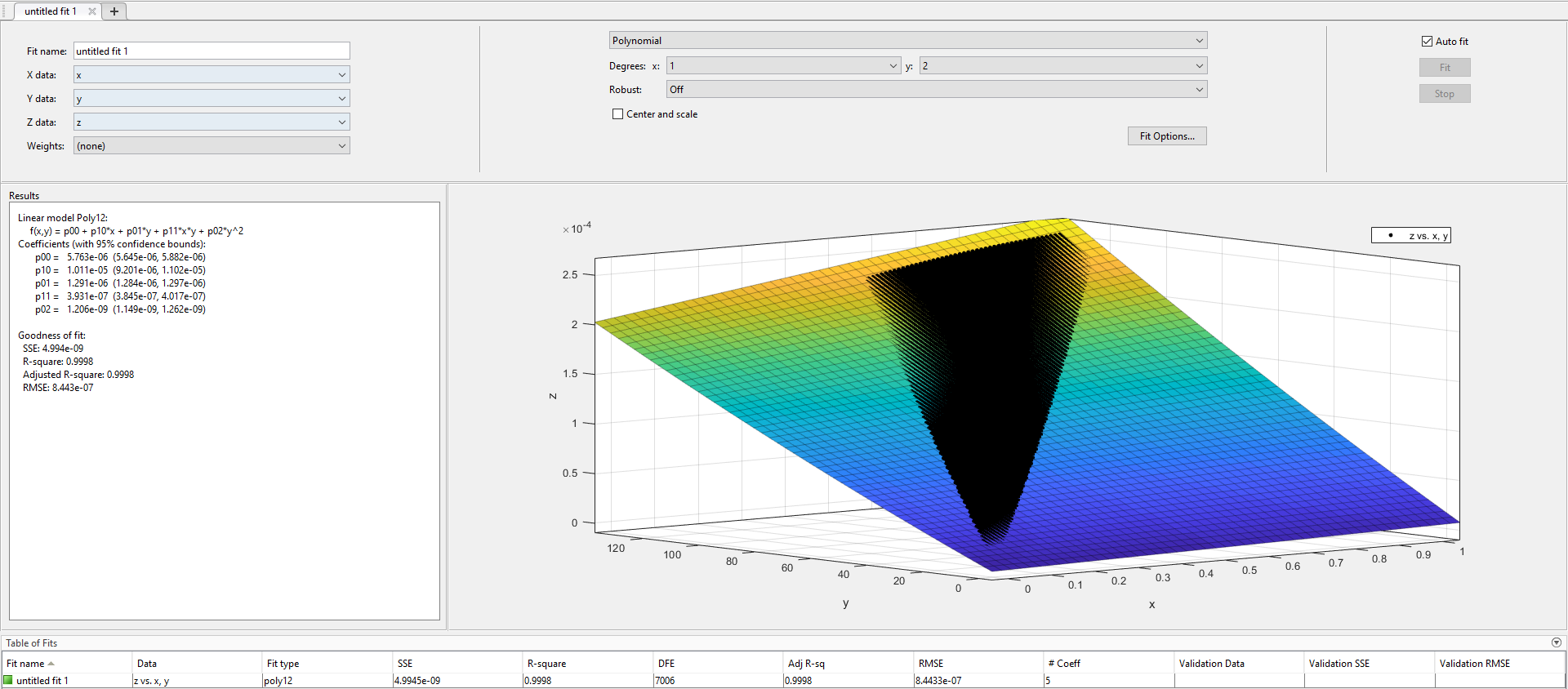if (I > 240*SOC_avg and <= 480*SOC_avg)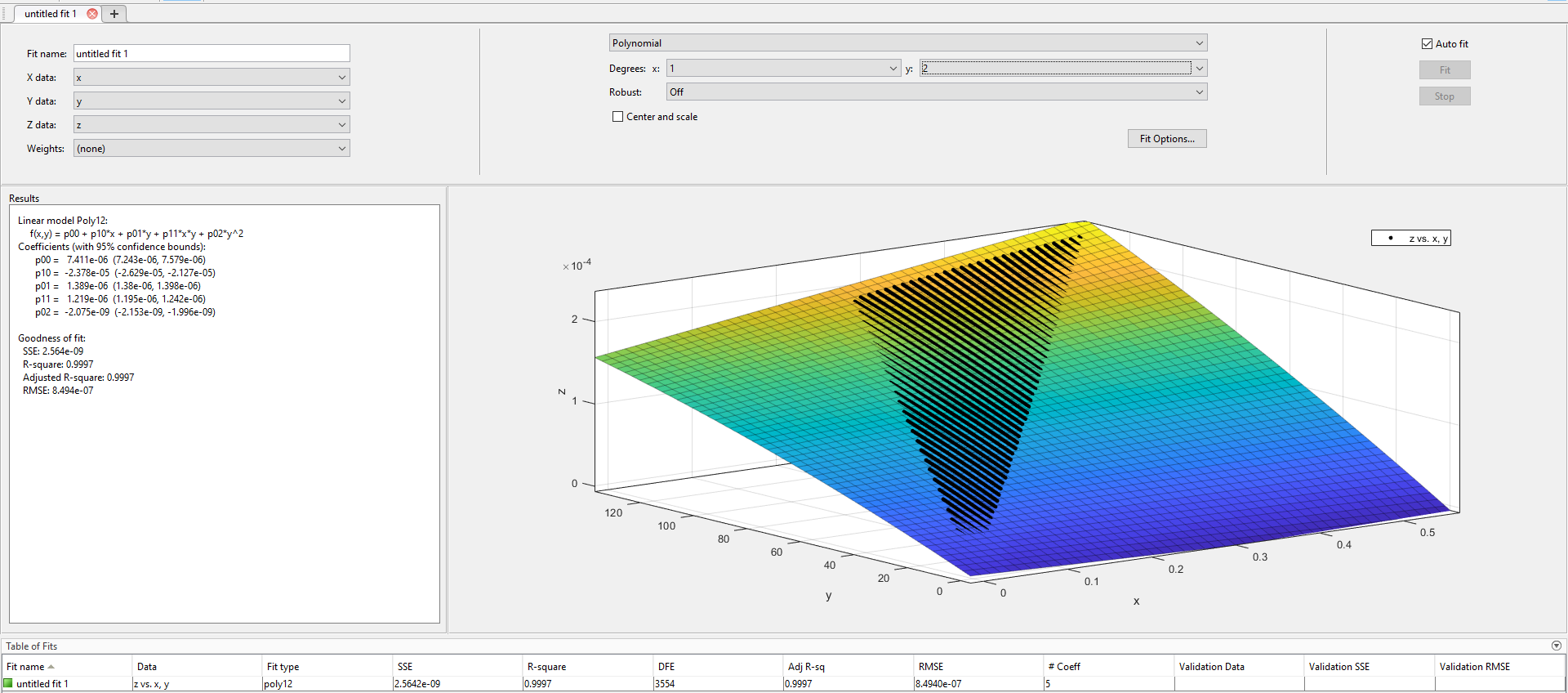if (I > 480*SOC_avg and <= 960*SOC_avg):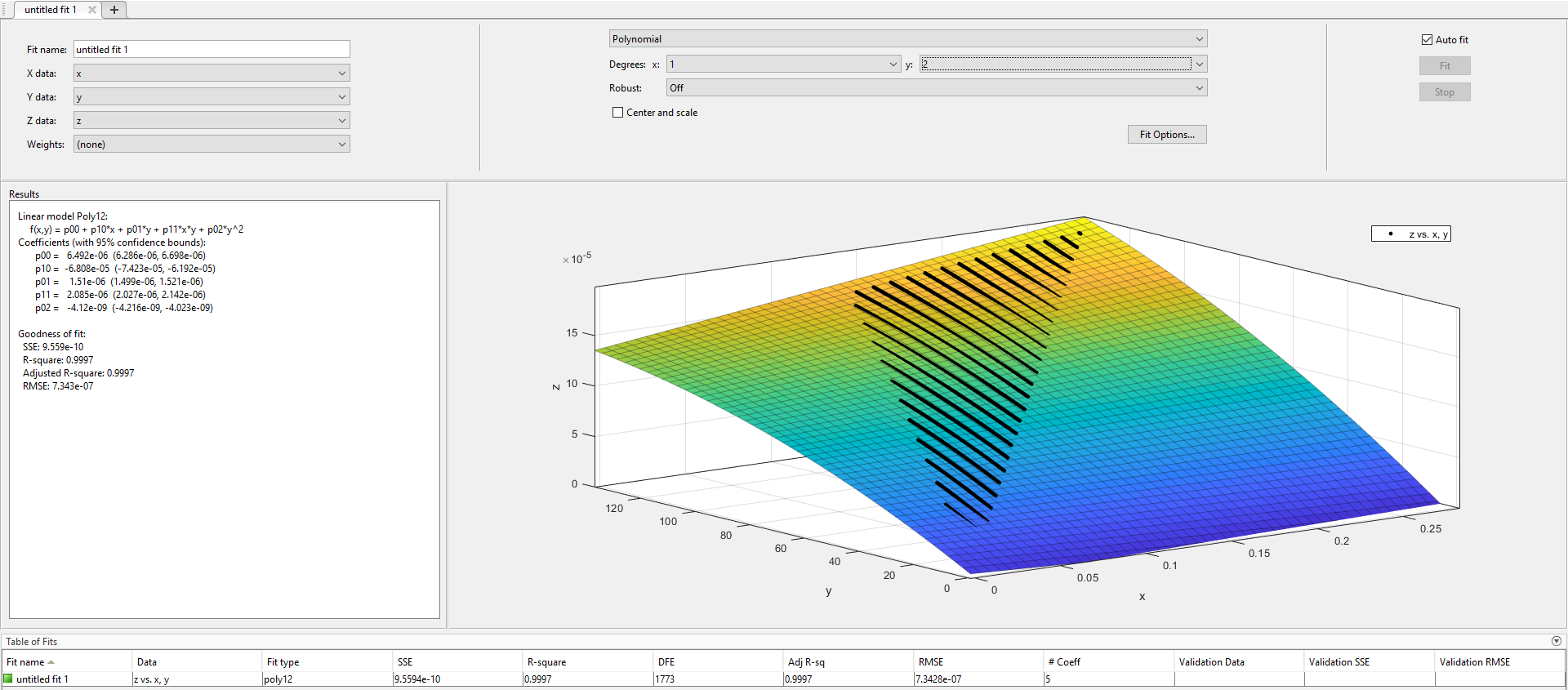if (I > 960*SOC_avg):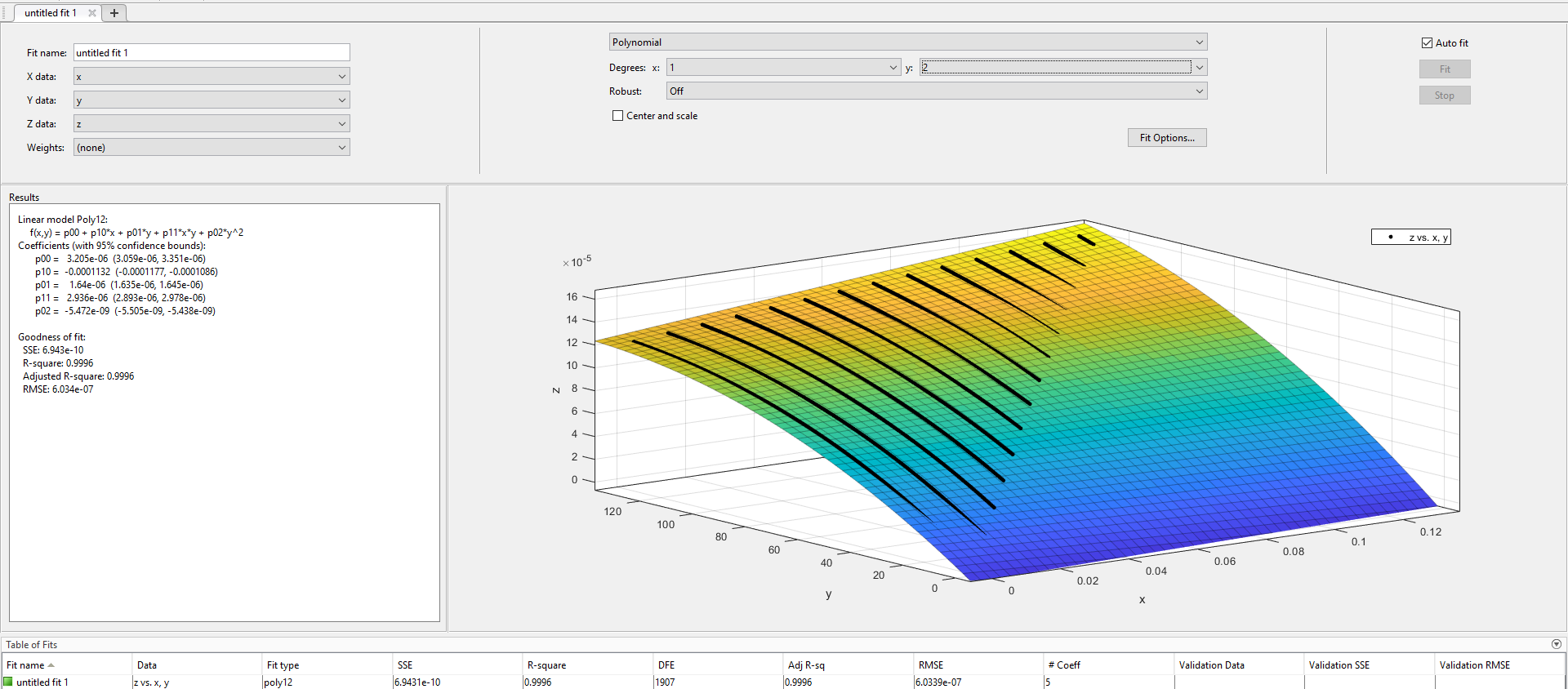I am trying to implement the conditional statement procedure. My code is given below. Could you please tell why I am get the following errors? Am I checking the binary variable correctly to choose my desired objective function?

    cap_loss = []    for v in range(0,Nv):        cap_loss.append( m.addVars((TT[v]), vtype=GRB.CONTINUOUS, lb=0, ub=0.05) )    for v in range(0,Nv):        for i in range(0,TT[v]):            # Model if x>y then b1 = 1, otherwise b1 = 0            m.addConstr(I[v][i] >= 960*SOC_avg[v][i] + eps - M * (1 - b1), name="bigM_constr1")            m.addConstr(I[v][i] <= 960*SOC_avg[v][i] + M * b1, name="bigM_constr16")            if (b1 == 0):                m.addConstr(I[v][i] >= 480*SOC_avg[v][i] + eps - M * (1 - b2), name="bigM_constr2")                print('\n\n 2nd objective \n\n')            elif (b2 == 0):                m.addConstr(I[v][i] >= 240*SOC_avg[v][i] + eps - M * (1 - b3), name="bigM_constr3")                print('\n\n 3nd objective \n\n')            elif (b3 == 0):                m.addConstr(I[v][i] >= 120*SOC_avg[v][i] + eps - M * (1 - b4), name="bigM_constr4")                m.addConstr(I[v][i] <= 120*SOC_avg[v][i] + M * b4, name="bigM_constr5")                print('\n\n 4th or 5th objective \n\n')                            m.addConstr((b1 == 1) >> (cap_loss[v][i] ==  p00b1 + p10b1*SOC_avg[v][i] + p01b1*(I[v][i]) + p11b1*SOC_avg[v][i]*(I[v][i]) + p02b1*(I[v][i])**2  + p1*SOC_avg[v][i]*adj_var + p2  + q1*(I[v][i]/Cbat[v])**2 + q2*(I[v][i]/Cbat[v]) + q3  ), name="indicator_constr1")            m.addConstr((b2 == 1) >> (cap_loss[v][i] ==   p00b2 + p10b2*SOC_avg[v][i] + p01b2*(I[v][i]) + p11b2*SOC_avg[v][i]*(I[v][i]) + p02b2*(I[v][i])**2  + p1*SOC_avg[v][i]*adj_var + p2  + q1*(I[v][i]/Cbat[v])**2 + q2*(I[v][i]/Cbat[v]) + q3  ), name="indicator_constr2")            m.addConstr((b3 == 1) >> (cap_loss[v][i] ==  p00b3 + p10b3*SOC_avg[v][i] + p01b3*(I[v][i]) + p11b3*SOC_avg[v][i]*(I[v][i]) + p02b3*(I[v][i])**2  + p1*SOC_avg[v][i]*adj_var + p2  + q1*(I[v][i]/Cbat[v])**2 + q2*(I[v][i]/Cbat[v]) + q3  ), name="indicator_constr3")            m.addConstr((b4 == 1) >> (cap_loss[v][i] ==  p00b4 + p10b4*SOC_avg[v][i] + p01b4*(I[v][i]) + p11b4*SOC_avg[v][i]*(I[v][i]) + p02b4*(I[v][i])**2  + p1*SOC_avg[v][i]*adj_var + p2  + q1*(I[v][i]/Cbat[v])**2 + q2*(I[v][i]/Cbat[v]) + q3  ), name="indicator_constr4")            m.addConstr((b4 == 0) >> (cap_loss[v][i] ==  p00b5 + p10b5*SOC_avg[v][i] + p01b5*(I[v][i]) + p11b5*SOC_avg[v][i]*(I[v][i]) + p02b5*(I[v][i])**2  + p1*SOC_avg[v][i]*adj_var + p2  + q1*(I[v][i]/Cbat[v])**2 + q2*(I[v][i]/Cbat[v]) + q3  ), name="indicator_constr5")
The error I am getting is as below: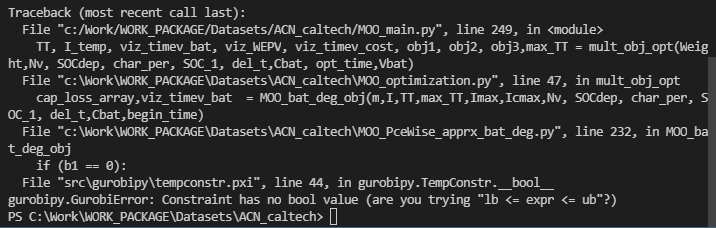when I try
if (b1.x != 1) or if (b1.x == 0)

I am still getting the following error.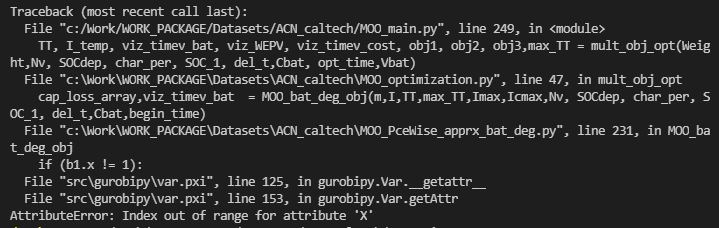Thanks again.

•Gurobi Staff

Hi Jose,

It seems you are trying to use the value of a variable directly in the formulation. You cannot have an if-condition as

if b1 == 0:

for a binary variable b1. You need to use indicator constraints to formulate this condition just like you do in the lines below.

Please note that you cannot query the value of a variable before the optimization has even started.

I hope that helps.

Cheers,
Matthias

•How do I specify disjoint intervals like
I> 960*SOC_avg ,
480*SOC_avg < I < 960*SOC_avg ,
240*SOC_avg < I < 480*SOC_avg ,
120*SOC_avg < I < 240*SOC_avg ,
and I < 240*SOC_avg

as a bigM constraint ?

Is there any notation I need to use in code?

Thanks.

•Gurobi Staff

Hi Jose,

Well, you might want to specify a binary variable for each segment/interval and then set up the corresponding indicator constraints to enforce your variables to be within these intervals.

Cheers,
Matthias

•Gurobi Staff

For example, say you have four different regions based on the relationship of x and y in which you have different objective functions. Then you need four binary variables to indicate in which region you are. Moreover, you will have four different objective functions that require the introduction of one auxiliary variable each. Note that because you are minimizing, it suffices to use an inequality to link the objective auxiliary variables to their objective expressions.

For example:

min objs.t.# link auxiliary objective variables to objective expressionsobj1 >= expression1(x,y)obj2 >= expression2(x,y)obj3 >= expression3(x,y)obj4 >= expression4(x,y)# we are in exactly one regionz1 + z2 + z3 + z4 = 1# define the relation of x and y in each regionz1 = 1 -> y <= 120*xz2 = 1 -> y >= 120*xz2 = 1 -> y <= 240*xz3 = 1 -> y >= 240*xz3 = 1 -> y <= 480*xz4 = 1 -> y >= 480*x# the overall objective function value should be the one of the region in which we arez1 = 1 -> obj >= obj1z2 = 1 -> obj >= obj2z3 = 1 -> obj >= obj3z4 = 1 -> obj >= obj4bounds0 <= x <= 10 <= y <= 120binariesz1 z2 z3 z4

Something like this should give you what you want. But the question is how easy this will be to solve. The more regions you have, the tougher the problem will be due to the combinatorial explosion.

Another approach would be to simply create one Gurobi model for each individual region and solve these independently (you can even parallelize this yourself if you have a machine with lots of cores, but make sure to use an own Gurobi environment for each thread that you create). Then, your final solution would be the one with the best objective value among all the solutions of your Gurobi models.

Regards,

Tobias

•Hi Tobias,

I have written this code following your instruction above. Could you please check if its right? I am now getting Model is Infeasible error.

    b1  = m.addVar(vtype=GRB.BINARY, name="b1")    b2  = m.addVar(vtype=GRB.BINARY, name="b2")    b3  = m.addVar(vtype=GRB.BINARY, name="b3")    b4  = m.addVar(vtype=GRB.BINARY, name="b4")    b5  = m.addVar(vtype=GRB.BINARY, name="b5")    eps = 0.0001    M = 120 + eps  # smallest possible given bounds on x and y
    cap_loss = []    for v in range(0,Nv):        cap_loss.append( m.addVars((TT[v]), vtype=GRB.CONTINUOUS, lb=0, ub=0.05) )        for v in range(0,Nv):        for i in range(0,TT[v]):            obj1 = m.addVar(vtype=GRB.CONTINUOUS, name="obj1")            obj2 = m.addVar(vtype=GRB.CONTINUOUS, name="obj2")            obj3 = m.addVar(vtype=GRB.CONTINUOUS, name="obj3")            obj4 = m.addVar(vtype=GRB.CONTINUOUS, name="obj4")            obj5 = m.addVar(vtype=GRB.CONTINUOUS, name="obj5")            m.addConstr( obj1, GRB.EQUAL, p00b1 + p10b1*SOC_avg[v][i] + p01b1*(I[v][i]) + p11b1*SOC_avg[v][i]*(I[v][i]) + p02b1*(I[v][i])**2  + p1*SOC_avg[v][i]*adj_var + p2  + q1*(I[v][i]/Cbat[v])**2 + q2*(I[v][i]/Cbat[v]) + q3 )            m.addConstr( obj2,GRB.EQUAL, p00b2 + p10b2*SOC_avg[v][i] + p01b2*(I[v][i]) + p11b2*SOC_avg[v][i]*(I[v][i]) + p02b2*(I[v][i])**2  + p1*SOC_avg[v][i]*adj_var + p2  + q1*(I[v][i]/Cbat[v])**2 + q2*(I[v][i]/Cbat[v]) + q3)            m.addConstr( obj3,GRB.EQUAL, p00b3 + p10b3*SOC_avg[v][i] + p01b3*(I[v][i]) + p11b3*SOC_avg[v][i]*(I[v][i]) + p02b3*(I[v][i])**2  + p1*SOC_avg[v][i]*adj_var + p2  + q1*(I[v][i]/Cbat[v])**2 + q2*(I[v][i]/Cbat[v]) + q3)            m.addConstr(obj4, GRB.EQUAL, p00b4 + p10b4*SOC_avg[v][i] + p01b4*(I[v][i]) + p11b4*SOC_avg[v][i]*(I[v][i]) + p02b4*(I[v][i])**2  + p1*SOC_avg[v][i]*adj_var + p2  + q1*(I[v][i]/Cbat[v])**2 + q2*(I[v][i]/Cbat[v]) + q3)            m.addConstr(obj5, GRB.EQUAL, p00b5 + p10b5*SOC_avg[v][i] + p01b5*(I[v][i]) + p11b5*SOC_avg[v][i]*(I[v][i]) + p02b5*(I[v][i])**2  + p1*SOC_avg[v][i]*adj_var + p2  + q1*(I[v][i]/Cbat[v])**2 + q2*(I[v][i]/Cbat[v]) + q3)            m.addConstr(  b1 + b2 + b3 + b4 + b5 == 1 , name="single_region_constr")            # Model if x>y then b1 = 1, otherwise b1 = 0            m.addConstr(I[v][i] <= 120*SOC_avg[v][i] + M * b1, name="bigM_constr1")            m.addConstr(I[v][i] >= 120*SOC_avg[v][i] + eps - M * (1 - b2), name="bigM_constr2")            m.addConstr(I[v][i] <= 240*SOC_avg[v][i] + M * b2, name="bigM_constr3")            m.addConstr(I[v][i] >= 240*SOC_avg[v][i] + eps - M * (1 - b3), name="bigM_constr3")            m.addConstr(I[v][i] <= 480*SOC_avg[v][i] + M * b3, name="bigM_constr4")            m.addConstr(I[v][i] >= 480*SOC_avg[v][i] + eps - M * (1 - b4), name="bigM_constr5")            m.addConstr(I[v][i] <= 960*SOC_avg[v][i] + M * b4, name="bigM_constr6")            m.addConstr(I[v][i] >= 960*SOC_avg[v][i] + eps - M * (1 - b5), name="bigM_constr7")                   m.addConstr((b5 == 1) >> (cap_loss[v][i] ==  obj1), name="indicator_constr1")            m.addConstr((b4 == 1) >> (cap_loss[v][i] ==   obj2  ), name="indicator_constr2")            m.addConstr((b3 == 1) >> (cap_loss[v][i] ==  obj3  ), name="indicator_constr3")            m.addConstr((b2 == 1) >> (cap_loss[v][i] ==  obj4  ), name="indicator_constr4")            m.addConstr((b1 == 1) >> (cap_loss[v][i] ==  obj5  ), name="indicator_constr5")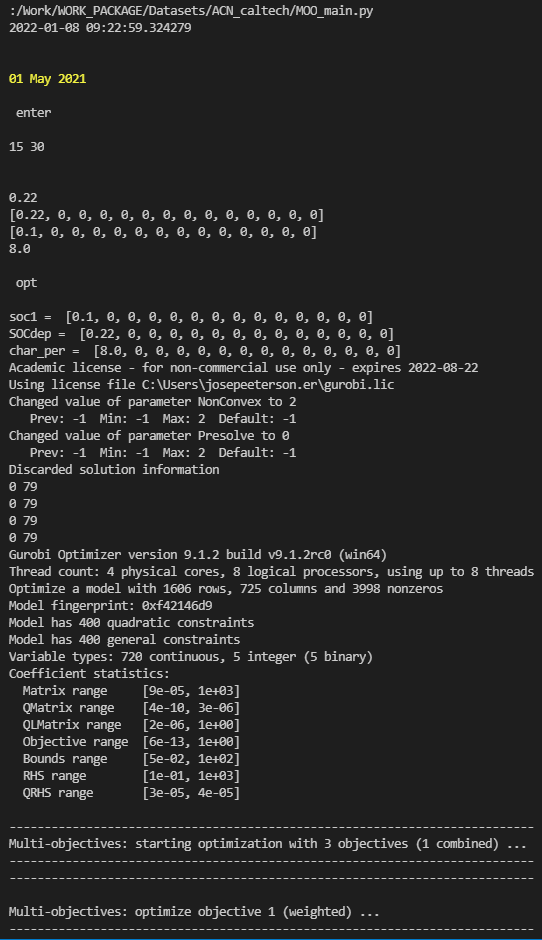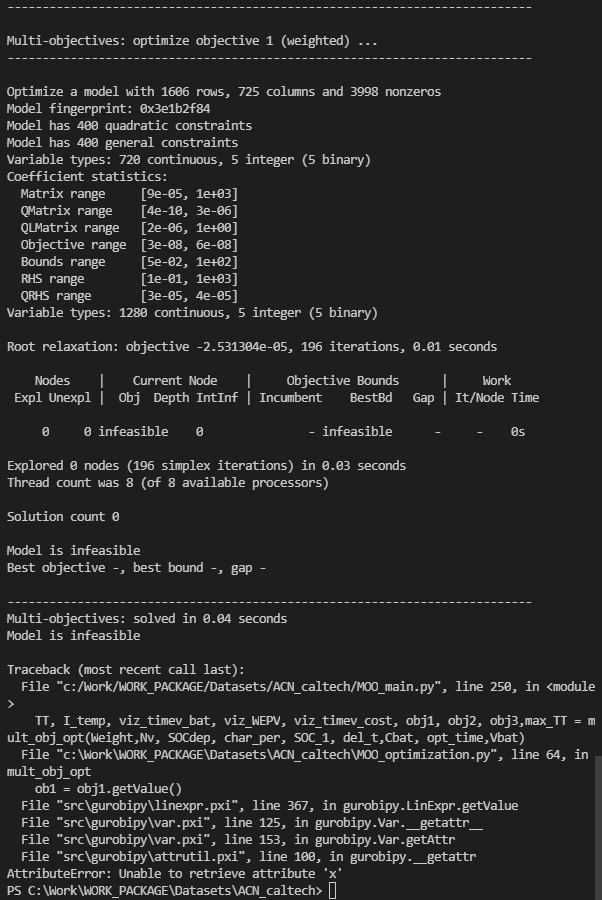•Gurobi Staff

I think that your big-M constraints are wrong. For example, b1=0 will force

I[v][i] <= 120*SOC_avg[v][i]

due to the first big-M constraint. This is not what you want. You only want to force constraint if the binary variable is 1. Why don't you just use the indicator constraints that I proposed as they are. Gurobi is supporting such indicator constraints directly. This is then easier to read as big-M constraints, and it may also have some advantage if Gurobi is able to find tighter big-Ms when it converts them into big-M during presolve.

Regards,

Tobias

•Gurobi Staff

In your formulation, I think that you always need to use (1 - bi) for all the big-M constraints as you have formulated them.

•Now my code looks like the way you mentioned. I want to makesure that the correct objective is chosen so I have written a small test code. When I run my code I am getting the following error.

import gurobipy as gpfrom gurobipy import GRBm = gp.Model('moo')m.params.NonConvex = 2m.params.Presolve = 0m.reset(0)b1  = m.addVar(vtype=GRB.BINARY, name="b1")b2  = m.addVar(vtype=GRB.BINARY, name="b2")b3  = m.addVar(vtype=GRB.BINARY, name="b3")b4  = m.addVar(vtype=GRB.BINARY, name="b4")b5  = m.addVar(vtype=GRB.BINARY, name="b5")#####   Paramters of calendric degradation     #####p1 =   5.387*10**-5p2 =   2.143*10**-5adj_var = 1 # adjustment variable to push the charging to later#####   Paramters of degradation due to current    #####q1 =  -0.0001528q2 =   0.0002643q3 =   1.134e-05#####   Paramters of cyclic degradation, piecewise  objectives   #####p00b1 = 3.205*10**-6    p10b1 = -0.0001132p01b1 = 1.64*10**-6p11b1 = 2.936*10**-6p02b1 = -5.472*10**-9p00b2 = 6.492*10**-6    p10b2 = -6.808*10**-5  p01b2 = 1.51*10**-6p11b2 = 2.085*10**-6p02b2 = -4.12*10**-9p00b3 = 7.411*10**-6    p10b3 = -2.378*10**-5  p01b3 = 1.389*10**-6p11b3 = 1.219*10**-6p02b3 = -2.075*10**-9p00b4 = 5.763*10**-6    p10b4 = 1.011*10**-5  p01b4 = 1.291*10**-6p11b4 = 3.931*10**7p02b4 = 1.206*10**-9p00b5 = 1.641*10**-6    p10b5 = -1.809*10**-6  p01b5 = 1.563*10**-6p11b5 = 2.6*10**-7p02b5 = 8.479*10**-10Nv = 1Cbat = *NvTT = SOC_avg = []I = []cap_loss = []for v in range(0,Nv):    cap_loss.append( m.addVars((TT[v]), vtype=GRB.CONTINUOUS, lb=0, ub=0.05) )for v in range(0,Nv):    for i in range(0,TT[v]):        obj1 = m.addVar(vtype=GRB.CONTINUOUS, name="obj1")        obj2 = m.addVar(vtype=GRB.CONTINUOUS, name="obj2")        obj3 = m.addVar(vtype=GRB.CONTINUOUS, name="obj3")        obj4 = m.addVar(vtype=GRB.CONTINUOUS, name="obj4")        obj5 = m.addVar(vtype=GRB.CONTINUOUS, name="obj5")        obj5 >= p00b1 + p10b1*SOC_avg[v][i] + p01b1*(I[v][i]) + p11b1*SOC_avg[v][i]*(I[v][i]) + p02b1*(I[v][i])**2  + p1*SOC_avg[v][i]*adj_var + p2  + q1*(I[v][i]/Cbat[v])**2 + q2*(I[v][i]/Cbat[v]) + q3        obj4 >= p00b2 + p10b2*SOC_avg[v][i] + p01b2*(I[v][i]) + p11b2*SOC_avg[v][i]*(I[v][i]) + p02b2*(I[v][i])**2  + p1*SOC_avg[v][i]*adj_var + p2  + q1*(I[v][i]/Cbat[v])**2 + q2*(I[v][i]/Cbat[v]) + q3        obj3 >= p00b3 + p10b3*SOC_avg[v][i] + p01b3*(I[v][i]) + p11b3*SOC_avg[v][i]*(I[v][i]) + p02b3*(I[v][i])**2  + p1*SOC_avg[v][i]*adj_var + p2  + q1*(I[v][i]/Cbat[v])**2 + q2*(I[v][i]/Cbat[v]) + q3        obj2 >= p00b4 + p10b4*SOC_avg[v][i] + p01b4*(I[v][i]) + p11b4*SOC_avg[v][i]*(I[v][i]) + p02b4*(I[v][i])**2  + p1*SOC_avg[v][i]*adj_var + p2  + q1*(I[v][i]/Cbat[v])**2 + q2*(I[v][i]/Cbat[v]) + q3        obj1 >= p00b5 + p10b5*SOC_avg[v][i] + p01b5*(I[v][i]) + p11b5*SOC_avg[v][i]*(I[v][i]) + p02b5*(I[v][i])**2  + p1*SOC_avg[v][i]*adj_var + p2  + q1*(I[v][i]/Cbat[v])**2 + q2*(I[v][i]/Cbat[v]) + q3        b1 + b2 + b3 + b4 + b5 == 1        # Model if x>y then b1 = 1, otherwise b1 = 0        b1 = 1 >> I[v][i] <= 120*SOC_avg[v][i]        b2 = 1 >> I[v][i] >= 120*SOC_avg[v][i]        b2 = 1 >> I[v][i] <= 240*SOC_avg[v][i]        b3 = 1 >> I[v][i] >= 240*SOC_avg[v][i]        b3 = 1 >> I[v][i] <= 480*SOC_avg[v][i]        b4 = 1 >> I[v][i] >= 480*SOC_avg[v][i]        b4 = 1 >> I[v][i] <= 960*SOC_avg[v][i]        b5 = 1 >> I[v][i] >= 960*SOC_avg[v][i]               (b5 == 1) >> (cap_loss[v][i] >=  obj5)        b4 == 1 >> cap_loss[v][i] >=  obj4        b3 == 1 >> cap_loss[v][i] >=  obj3        b2 == 1 >> cap_loss[v][i] >=  obj2        b1 == 1 >> cap_loss[v][i] >=  obj1cap_loss_array = []for v in range(0,Nv):    for i in range(0,TT[v]):        cap_loss_array.append(cap_loss[v][i])m.setObjective( sum( cap_loss_array))m.update()m.optimize()print(b1.x,b2.x,b3.x,b4.x,b5.x)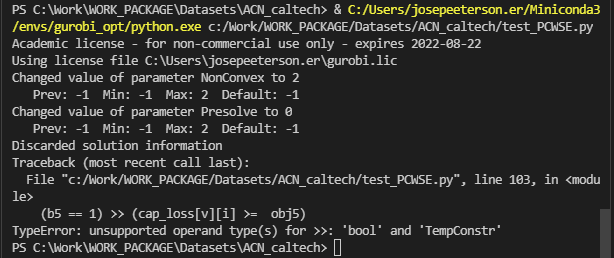I changed the syntax as below and still got these errors.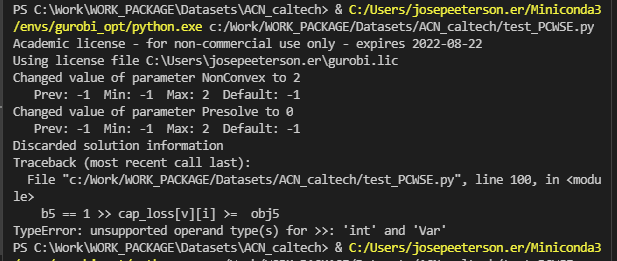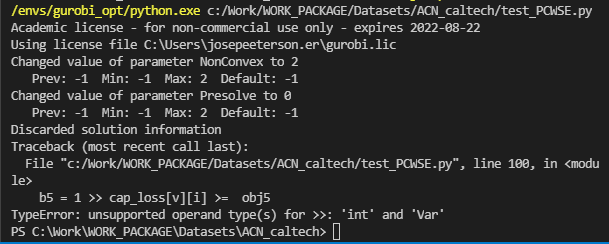Thanks.

•Gurobi Staff

•I have modified the indicator constraints using m.addGenConstrIndicator()

Now, it is choosing the regions correctly. However, If the number of decision variables are large the convergence is too slow. Is there any way to quicken the time to optimize apart from using fewer decision variables and reducing the number of piecewise regions?

The input to the optimization problem is given below.

del_t = 1##################    TESTINF FOR WORST CASE TIME TO SOLVE    #####################SOCdep =   [0.8, 0.8, 0.8, 0.8, 0.8, 0.8, 0.8]SOC_1 =    [0.1, 0.1, 0.1, 0.1, 0.1, 0.1, 0.1]char_per = [4,  4,  4,  4,  4,  4,  4]##################    TESTINF FOR WORST CASE TIME TO SOLVE    #####################

The number of elements in SOCdep represent the number of vehicles at a charging station. The value inside represents required final level of charge (0-1).  SOC_1 is the intial amoutnt of charge the vehicle has when it enters the charging station. char_per (charing period) is the time in hours for which a particular vehicle will charge. del_t is the time in hours that what will split each char_per into in this case 4/1 = 4 slots.

we will supply a specific current I[v][i] to charge a particular v in each of these 4 slots. This leads to 4*7 = 28 decision variables. However, I prefer a finer timeslot e.g. del_t = 0.1 hours then there will be 280 decisions variables. It takes ~5 mins to find teh solution. I would like real-time performace in under 30 seconds.  I am currently thinking of making del_t adapative for each vehicle depending on a short or long char_per. This will kind of require me to do some code overhaul. :(

Could you suggest anyway to speed-up the optimization process? Currenly, I have resorted to using 3 piecewise regions instead of 5.

import gurobipy as gpfrom gurobipy import GRBimport math  import datetimem = gp.Model('moo')m.params.NonConvex = 2m.params.Presolve = 0m.reset(0)#####   Paramters of calendric degradation     #####p1 =   5.387*10**-5p2 =   2.143*10**-5adj_var = 1 # adjustment variable to push the charging to later#####   Paramters of degradation due to current    #####q1 =  -0.0001528q2 =   0.0002643q3 =   1.134e-05#####   Paramters of cyclic degradation, piecewise  objectives   #####p00b1 = 3.205*10**-6    p10b1 = -0.0001132p01b1 = 1.64*10**-6p11b1 = 2.936*10**-6p02b1 = -5.472*10**-9p00b2 = 6.492*10**-6    p10b2 = -6.808*10**-5  p01b2 = 1.51*10**-6p11b2 = 2.085*10**-6p02b2 = -4.12*10**-9p00b3 = 7.411*10**-6    p10b3 = -2.378*10**-5  p01b3 = 1.389*10**-6p11b3 = 1.219*10**-6p02b3 = -2.075*10**-9p00b4 = 5.763*10**-6    p10b4 = 1.011*10**-5  p01b4 = 1.291*10**-6p11b4 = 3.931*10**-7p02b4 = 1.206*10**-9p00b5 = 1.641*10**-6    p10b5 = -1.809*10**-6  p01b5 = 1.563*10**-6p11b5 = 2.6*10**-7p02b5 = 8.479*10**-10del_t = 1##################    TESTINF FOR WORST CASE TIME TO SOLVE    #####################SOCdep =   [0.8, 0.8, 0.8, 0.8, 0.8, 0.8, 0.8]SOC_1 =    [0.1, 0.1, 0.1, 0.1, 0.1, 0.1, 0.1]char_per = [4,  4,  4,  4,  4,  4,  4]##################    TESTINF FOR WORST CASE TIME TO SOLVE    #####################start_time = datetime.datetime.now()SOC_xtra = 0.001t_s = 0Nv = len(SOCdep)Cbat = *NvTT = []for v in range(0,Nv):    TT.append( math.ceil((char_per[v] + t_s) / del_t) )max_TT = max(TT)Imax = 120Icmax = Imax*NvI = []for v in range(0,Nv):    I.append( m.addVars((TT[v]), vtype=GRB.CONTINUOUS, lb=0, ub=Imax) )# upper_bound battery power constraintbat_pwr_constr_l = []for v in range(0,Nv):    for i in range(0,TT[v]):        bat_pwr_constr_l.append( m.addConstr( I[v][i] >= 0 ) )# lower_bound battery power constraintbat_pwr_constr_u = []for v in range(0,Nv):    for i in range(0,TT[v]):        bat_pwr_constr_u.append( m.addConstr( I[v][i] <= Imax ) )# slot power constraintfor i in range(0,max_TT):    slot_pwr = []    for v in range(0,Nv):        if i < TT[v]:            slot_pwr.append(I[v][i])    m.addConstr( sum(slot_pwr) <= Icmax )# lower_bound Energy constrainttot_char_curr = []for v in range(0,Nv):    each_veh_curr = []    for i in range(0,TT[v]):        each_veh_curr.append(I[v][i])        tot_char_curr.append(I[v][i])    if(TT[v] > 0):        m.addConstr( ( sum(each_veh_curr) )* del_t  >= (SOCdep[v] - SOC_1[v])*Cbat[v] )        print(v,i)# upper_bound Energy constraintfor v in range(0,Nv):    each_veh_curr = []    for i in range(0,TT[v]):        each_veh_curr.append(I[v][i])    if(TT[v] > 0):        m.addConstr( ( sum(each_veh_curr) )* del_t  <= (SOCdep[v] - SOC_1[v] + SOC_xtra)*Cbat[v]  )#like boby variable in smaple code.SOC = []for v in range(0,Nv):    SOC.append( m.addVars((TT[v]), vtype=GRB.CONTINUOUS, lb=0, ub=1) )#constraint updatefor v in range(0,Nv):    first = 1    for i in range(0,TT[v]):        if first == 1:            m.addConstr(SOC[v][i], GRB.EQUAL, SOC_1[v] + (I[v][i]* del_t) / Cbat[v])            first = 0        else:            m.addConstr(SOC[v][i], GRB.EQUAL, SOC[v][i-1] + (I[v][i]* del_t) / Cbat[v])SOC_avg = []for v in range(0,Nv):    SOC_avg.append( m.addVars((TT[v]), vtype=GRB.CONTINUOUS, lb=0, ub=1) )# SOC constraint updatefor v in range(0,Nv):    first = 1    for i in range(0,TT[v]):        if first == 1:            m.addConstr(SOC_avg[v][i], GRB.EQUAL, SOC_1[v] + (0.5*(I[v][i]* del_t)) / Cbat[v])            first = 0        else:                    m.addConstr(SOC_avg[v][i], GRB.EQUAL, SOC[v][i-1] + (0.5*(I[v][i]* del_t)) / Cbat[v])b1 = []b2 = []b3 = []b4 = []b5 = []for v in range(0,Nv):    # b1.append( m.addVars((TT[v]),vtype=GRB.BINARY) )    # b2.append( m.addVars((TT[v]),vtype=GRB.BINARY) )    b3.append( m.addVars((TT[v]),vtype=GRB.BINARY) )    b4.append( m.addVars((TT[v]),vtype=GRB.BINARY) )    b5.append( m.addVars((TT[v]),vtype=GRB.BINARY) )cap_loss = []for v in range(0,Nv):    cap_loss.append( m.addVars((TT[v]), vtype=GRB.CONTINUOUS, lb=0, ub=0.05) )for v in range(0,Nv):    for i in range(0,TT[v]):        # b1  = m.addVar(vtype=GRB.BINARY)        # b2  = m.addVar(vtype=GRB.BINARY)        # b3  = m.addVar(vtype=GRB.BINARY)        # b4  = m.addVar(vtype=GRB.BINARY)        # b5  = m.addVar(vtype=GRB.BINARY)        obj1 = m.addVar(vtype=GRB.CONTINUOUS, name="obj1")        obj2 = m.addVar(vtype=GRB.CONTINUOUS, name="obj2")        obj3 = m.addVar(vtype=GRB.CONTINUOUS, name="obj3")        obj4 = m.addVar(vtype=GRB.CONTINUOUS, name="obj4")        obj5 = m.addVar(vtype=GRB.CONTINUOUS, name="obj5")        m.addConstr( obj5, GRB.EQUAL, p00b1 + p10b1*SOC_avg[v][i] + p01b1*(I[v][i]) + p11b1*SOC_avg[v][i]*(I[v][i]) + p02b1*(I[v][i])**2  + p1*SOC_avg[v][i]*adj_var + p2 )# + q1*(I[v][i]/Cbat[v])**2 + q2*(I[v][i]/Cbat[v]) + q3 )        m.addConstr( obj4,GRB.EQUAL, p00b2 + p10b2*SOC_avg[v][i] + p01b2*(I[v][i]) + p11b2*SOC_avg[v][i]*(I[v][i]) + p02b2*(I[v][i])**2  + p1*SOC_avg[v][i]*adj_var + p2 )#  + q1*(I[v][i]/Cbat[v])**2 + q2*(I[v][i]/Cbat[v]) + q3)        m.addConstr( obj3,GRB.EQUAL, p00b3 + p10b3*SOC_avg[v][i] + p01b3*(I[v][i]) + p11b3*SOC_avg[v][i]*(I[v][i]) + p02b3*(I[v][i])**2  + p1*SOC_avg[v][i]*adj_var + p2 )#  + q1*(I[v][i]/Cbat[v])**2 + q2*(I[v][i]/Cbat[v]) + q3)        # m.addConstr(obj2, GRB.EQUAL, p00b4 + p10b4*SOC_avg[v][i] + p01b4*(I[v][i]) + p11b4*SOC_avg[v][i]*(I[v][i]) + p02b4*(I[v][i])**2  + p1*SOC_avg[v][i]*adj_var + p2 )#  + q1*(I[v][i]/Cbat[v])**2 + q2*(I[v][i]/Cbat[v]) + q3)        # m.addConstr(obj1, GRB.EQUAL, p00b5 + p10b5*SOC_avg[v][i] + p01b5*(I[v][i]) + p11b5*SOC_avg[v][i]*(I[v][i]) + p02b5*(I[v][i])**2  + p1*SOC_avg[v][i]*adj_var + p2 )#  + q1*(I[v][i]/Cbat[v])**2 + q2*(I[v][i]/Cbat[v]) + q3)        m.addConstr( b3[v][i] + b4[v][i] + b5[v][i], GRB.EQUAL, 1 ) # b1[v][i] + b2[v][i] + b3[v][i] + b3[v][i] +        # m.addGenConstrIndicator(b1[v][i], True, I[v][i] <= 120*SOC_avg[v][i])        # m.addGenConstrIndicator(b2[v][i], True, I[v][i] >= 120*SOC_avg[v][i])        # m.addGenConstrIndicator(b2[v][i], True, I[v][i] <= 240*SOC_avg[v][i])        # m.addGenConstrIndicator(b3[v][i], True, I[v][i] >= 240*SOC_avg[v][i])        m.addGenConstrIndicator(b3[v][i], True, I[v][i] <= 480*SOC_avg[v][i])        m.addGenConstrIndicator(b4[v][i], True, I[v][i] >= 480*SOC_avg[v][i])        m.addGenConstrIndicator(b4[v][i], True, I[v][i] <= 960*SOC_avg[v][i])        m.addGenConstrIndicator(b5[v][i], True, I[v][i] >= 960*SOC_avg[v][i])                       m.addConstr((b5[v][i] == 1) >> (cap_loss[v][i] ==  obj5), name="indicator_constr1")        m.addConstr((b4[v][i] == 1) >> (cap_loss[v][i] ==   obj4  ), name="indicator_constr2")        m.addConstr((b3[v][i] == 1) >> (cap_loss[v][i] ==  obj3  ), name="indicator_constr3")        # m.addConstr((b2[v][i] == 1) >> (cap_loss[v][i] ==  obj2  ), name="indicator_constr4")        # m.addConstr((b1[v][i] == 1) >> (cap_loss[v][i] ==  obj1  ), name="indicator_constr5")       cap_loss_array = []for v in range(0,Nv):    for i in range(0,TT[v]):        cap_loss_array.append(cap_loss[v][i])m.setObjective( sum( cap_loss_array),GRB.MINIMIZE)m.update()m.optimize()for v in range(0,Nv):    for i in range(0,TT[v]):        print(I[v][i].x)        print(SOC_avg[v][i].x)        #print(b1.x,b2.x,b3.x,b4.x,b5.x)        print(b3[v][i].x,b4[v][i].x,b5[v][i].x) #b1[v][i].x,b2[v][i].x,b3[v][i].x,print('\ntime elapsed = ', datetime.datetime.now() - start_time )

Thanks.

•Gurobi Staff

Please note that you turned off presolve by setting

m.params.Presolve = 0

If you turn it back on, i.e., remove this line, your problem solves within a second even with 5 objective pieces.

Best regards,
Jaromił

•Hi Jaromil,

Unfortunately, It does not solve the problem.

You can try to comment m.params.Presolve = 0 and change del_t from 1 to 0.1 It still takes very long to solve.

I am afraid I cannot use adaptive timeslot size as it will affect another objective in my multi-objective problem. so if you know any solutions, do let me know.

Thanks.

•Gurobi Staff

Hi Jose,

By setting $$\texttt{del_t=0.1}$$, you increase the number of variables and constraints by a factor of 10. Given that your model is nonconvex and additionally uses a lot of piecewise linear constraints, it is not surprising that the solution time increases exponentially.

In this situation, it might be best to try to find a good tradeoff between the $$\texttt{del_t}$$ value and the number of pieces used for the approximation of the objective function.

Then, there is also the fact, that most of the coefficients you use are very small. Many of the coefficients are even below the lowest feasibility tolerance possible, cf. FeasibilityTol. If I understand correctly, these coefficients are used to approximate the objective function. You should definitely reconsider the quality of the approximation of the objective function to avoid possible numerical difficulties, cf. Guidelines for Numerical Issues. It might make sense to drop very small coefficients even if the approximation quality decreases in order to improve the overall performance. This is something that might be worth trying out.

An alternative which might be worth exploring is to solve the model on each objective approximation part independently instead of constructing one model with 5 objective approximations. This might help in identifying an approximation region which is for some reason harder to solve than the others. With this information, you can then try to chose the approximation regions differently.

In your first post, you stated that the objective function is defined by an exponential and many products. Did you actually try modeling the objective function with the addGenConstrExp method and additional product terms? Large part of the objective function looks linear. It might be worth to try to approximate one part of the objective function with an $$\exp$$ function and the other linearly.

Best regards,
Jaromił

•Hi Jaromil,

I have now resorted to use del_t = 0.25. I have also chosen to use only 2 piecewise objectives. I am also using presolve commented out. Now, I am able to get near real-time performance. :)

After the approximation, I just have a simple 2nd order polynomial so I should be able to scale it up by mulpliplying with a suitable factor e.g. 1000.

I don't quite understand ' solve the model on each objective approximation part independently instead of constructing one model with 5 objective approximations. ' since these objectives are only active in certain domains. It is possible that a wrong objective outside its domain will give a lower value than the right objective due to bad approximations outside the wrong objective's domain. If you would like clarify this point a little more that will be helpful. Thanks. :)

I tried implementing the actual equation it was taking too long to solve.

Thanks Jaromil and the Gurobi team for your dedicated support.

•Gurobi Staff

Hi Jose,

I don't quite understand ' solve the model on each objective approximation part independently instead of constructing one model with 5 objective approximations. ' since these objectives are only active in certain domains. It is possible that a wrong objective outside its domain will give a lower value than the right objective due to bad approximations outside the wrong objective's domain. If you would like clarify this point a little more that will be helpful. Thanks. :)

The idea would be to solve 2 models. One with $$y\geq 120x$$ and the other with $$y\leq 120x$$. This would reduce the problem size of each model but you would have to choose the correct solution in the end. It might also happen that one of these models is infeasible, so I guess it is best to approach this problem in the way you used in your last message.

I tried implementing the actual equation it was taking too long to solve.

Ok.

Best regards,
Jaromił